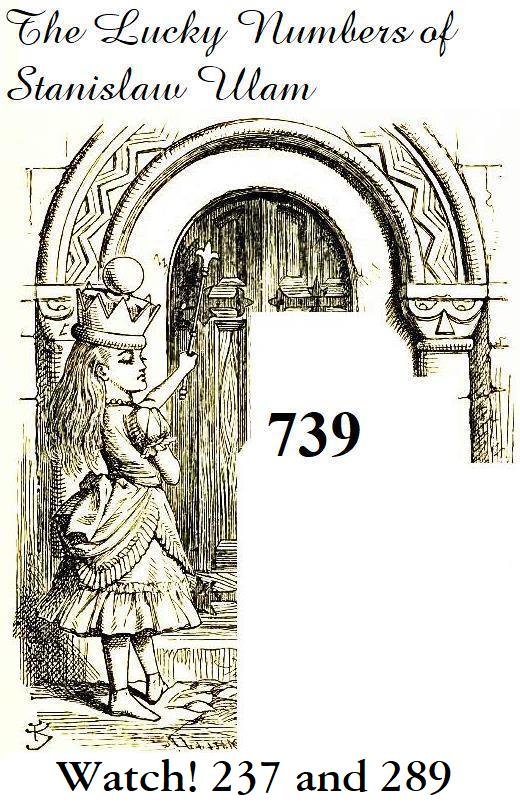[Previous] [Up] [Home] [Other] [Mathematics]The prine numbers, of course, are produced by means of the Sieve of Erastothenes.

While working at Los Alamos, Dr. Stanislaw Ulam concieved the idea of creating an alternative series of numbers, produced by means of a different sieve which resembled, but was not identical with, the Sieve of Erastothenes.

As this sieve somewhat resembled the method of elimination in what is known as the Josephus problem, it seemed appropriate to call the surviving numbers "lucky". As the sieve operated within the remaining numbers, and not all numbers, it would be reasonable to expect the "lucky numbers" to be somewhat less random in their distribution than the primes.

And, therefore, it might be that they would prove useful in investigating the Prime Number Theorem, particularly as it relates to the Riemann Conjecture.

The Sieve of Eratosthenes works like this:

``` 2  3  4  5  6  7  8  9 10 11 12 13 14 15 16 17 18 19 20 21 22 23 24 25 26 27 28 29 30 31 32 33
2     x     x     x     x     x     x     x     x     x     x     x     x     x     x     x
3        x        x        x        x        x        x        x        x        x        x
5              x              x              x              x              x
7                    x                    x                    x
11                                x                                x
```

where, once a number is acknowledged as a prime, all its multiples are sieved out, and the sieve for the lucky numbers works like this:

``` 1  2  3  4  5  6  7  8  9 10 11 12 13 14 15 16 17 18 19 20 21 22 23 24 25 26 27 28 29 30 31 32 33
x     x     x     x     x     x     x     x     x     x     x     x     x     x     x     x
3     x                 x                 x                 x                 x
7                                   x
```

In the first step, starting from 1, every second number is eliminated; here, this means that 2 is eliminated, rather than taken as a lucky number. Note that 1 is considered to be a lucky number.

In the second step, again starting from 1, every third number among those remaining is eliminated; this leaves 3 as the next luky number, eliminating 5, 11, 17, 23, and 29 and so on.

After the second step, the next lowest remaining number is 7, so every seventh number among those remaining, starting from 1, is eliminated, which this time starts with 19, and so on.

[Previous] [Up] [Home] [Other] [Mathematics]